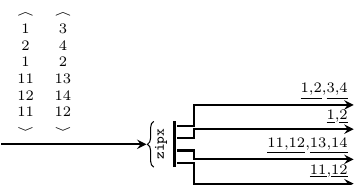forsyde-atom-0.3.0.0: Shallow-embedded DSL for modeling cyber-physical systems

ForSyDe.Atom.MoC.SDF

Description

The SDF library implements a DSL of atoms operating according to the synchronous dataflow model of computation, along with helpers and associated patterns.

There are many kinds of dataflow (DF) MoCs found in literature, many of which extend the original SDF formulation of [Lee87]. ForSyDe-Atom implements some of the common SDF extensions (see below), and this module exports the core atoms for all of them. As compared to other MoC sub-modules (see ForSyDe.Atom.SY.Clocked,ForSyDe.Atom.DE.React), the SDF sub-modules define only new patterns describing meaningful processes according to each DF class. The common atom semantics imply a partial order between events and operate on sequences of events, here represented with lists. As a consequence, we use the same event constructor to identify any kind of DF signal, respectively we need no MoC interface between DF domains.

Useful pointers:

Synopsis

# Synchronous data flow (SDF) event

The synchronous data flow (SDF) is an untimed MoC. On untimed MoCs, [Lee98] states that: "when tags are partially ordered rather than totally ordered, we say that the system is untimed. Untimed systems cannot have the same notion of causality as timed systems [see SY]. (...) Processes defined in terms of constraints on the tags in the signals (...) have a consistent cut rather than simultaneity." Regarding SDF, it states that "is a special case of Kahn process networks [Kahn76]. A dataflow process is a Kahn process that is also sequential, where the events on the self-loop signal denote the firings of the dataflow actor. The firing rules of a dataflow actor are partial ordering constraints between these events and events on the inputs. (...) Produced/consumed events are defined in terms of relations with the events in the firing signal. It results that for the same firing $$i$$, $$e_i<e_o$$, as an intuitive sort of causality constraint."

A simplified definition of the ForSyDe-Atom implementation of SDF is:

The SDF MoC
is abstracting the execution semantics of a dataflow system where computation is performed according to firing rules where the production and the consumption rates are fixed.

Below is a depiction of the the input and the output behavior of a SDF process. Events sharing the same partial ordering in relation to a certain firing are underlined: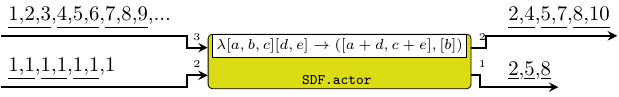Implementing the SDF tag system implied a series of design decisions which lead to the following particularities:

1. signals represent FIFO channels, and tags are implicit from their position in the Stream structure. Internally, SDF signals have exactly the same structure as SY signals, whereas the partial ordering is imposed by the processes alone.
2. the SDF event constructor wraps only a value.
3. the order between events is partial to the firings of processes. An SDF atom will fire only when there are enough events to trigger its inputs. Once a firing occurs, it will take care of partitioning the input or output signals.
4. SDF atoms require a context for executing the passed functions: the consumption $$c$$ and production $$p$$ rates need to be known in order to determine the behavior of a process.
5. the previous statement can be synthesized into the following formal definition for the SDF execution context: $\Gamma\vdash\alpha\rightarrow\beta = (c,p,[\alpha]_c\rightarrow[\beta]_p)$ where the indexes $$_c$$ and $$_p$$ suggest the mandatory lengths of the sequences of $$\alpha$$ and $$\beta$$ respectively. In our case, due to language limitations, $$c$$ and $$p$$ are passed as context arguments since they cannot be inferred from the type signature.

To see how ForSyDe-Atom implements the above definition in practice, check the type family instance for the Fun and Ret types.

newtype SDF a Source #

The SDF event. It identifies a synchronous dataflow signal, and wraps only a value.

Constructors

 SDF Fieldsval :: a

#### Instances

Instances details
 Source # Allows for mapping of functions on a SDF event. Instance detailsDefined in ForSyDe.Atom.MoC.SDF.Core Methodsfmap :: (a -> b) -> SDF a -> SDF b #(<\$) :: a -> SDF b -> SDF a # Source # Allows for lifting functions on a pair of SDF events. Instance detailsDefined in ForSyDe.Atom.MoC.SDF.Core Methodspure :: a -> SDF a #(<*>) :: SDF (a -> b) -> SDF a -> SDF b #liftA2 :: (a -> b -> c) -> SDF a -> SDF b -> SDF c #(*>) :: SDF a -> SDF b -> SDF b #(<*) :: SDF a -> SDF b -> SDF a # Source # Instance detailsDefined in ForSyDe.Atom.MoC.SDF.Core Methodsfold :: Monoid m => SDF m -> m #foldMap :: Monoid m => (a -> m) -> SDF a -> m #foldMap' :: Monoid m => (a -> m) -> SDF a -> m #foldr :: (a -> b -> b) -> b -> SDF a -> b #foldr' :: (a -> b -> b) -> b -> SDF a -> b #foldl :: (b -> a -> b) -> b -> SDF a -> b #foldl' :: (b -> a -> b) -> b -> SDF a -> b #foldr1 :: (a -> a -> a) -> SDF a -> a #foldl1 :: (a -> a -> a) -> SDF a -> a #toList :: SDF a -> [a] #null :: SDF a -> Bool #length :: SDF a -> Int #elem :: Eq a => a -> SDF a -> Bool #maximum :: Ord a => SDF a -> a #minimum :: Ord a => SDF a -> a #sum :: Num a => SDF a -> a #product :: Num a => SDF a -> a # Source # Instance detailsDefined in ForSyDe.Atom.MoC.SDF.Core Methodstraverse :: Applicative f => (a -> f b) -> SDF a -> f (SDF b) #sequenceA :: Applicative f => SDF (f a) -> f (SDF a) #mapM :: Monad m => (a -> m b) -> SDF a -> m (SDF b) #sequence :: Monad m => SDF (m a) -> m (SDF a) # Source # Implenents the SDF semantics for the MoC atoms. Instance detailsDefined in ForSyDe.Atom.MoC.SDF.Core Associated Typestype Fun SDF a b Source #type Ret SDF b Source # Methods(-.-) :: Fun SDF a b -> Stream (SDF a) -> Stream (SDF b) Source #(-*-) :: Stream (SDF (Fun SDF a b)) -> Stream (SDF a) -> Stream (SDF b) Source #(-*) :: Stream (SDF (Ret SDF b)) -> Stream (SDF b) Source #(-<-) :: Stream (SDF a) -> Stream (SDF a) -> Stream (SDF a) Source #(-&-) :: Stream (SDF a) -> Stream (SDF a) -> Stream (SDF a) Source # Read a => Read (SDF a) Source # Reads the value wrapped Instance detailsDefined in ForSyDe.Atom.MoC.SDF.Core MethodsreadsPrec :: Int -> ReadS (SDF a) #readList :: ReadS [SDF a] #readPrec :: ReadPrec (SDF a) # Show a => Show (SDF a) Source # Shows the value wrapped Instance detailsDefined in ForSyDe.Atom.MoC.SDF.Core MethodsshowsPrec :: Int -> SDF a -> ShowS #show :: SDF a -> String #showList :: [SDF a] -> ShowS # Plottable a => Plot (Signal a) Source # For plotting SDF signals. Instance detailsDefined in ForSyDe.Atom.Utility.Plot Methodssample :: Float -> Signal a -> Samples Source #takeUntil :: Float -> Signal a -> Signal a Source # type Ret SDF a Source # Instance detailsDefined in ForSyDe.Atom.MoC.SDF.Core type Ret SDF a = (Prod, [a]) type Fun SDF a b Source # Instance detailsDefined in ForSyDe.Atom.MoC.SDF.Core type Fun SDF a b = (Cons, [a] -> b)

# Aliases & utilities

These are type synonyms and utilities provided for user convenience. They mainly concern the construction and usage of signals.

type Signal a = Stream (SDF a) Source #

Type synonym for a SY signal, i.e. "a signal of SY events"

type Prod = Int Source #

Type synonym for consumption rate

type Cons = Int Source #

Type synonym for production rate

signal :: [a] -> Signal a Source #

Transforms a list of values into a SDF signal with only one partition, i.e. all events share the same (initial) tag.

Reads a signal from a string. Like with the read function from Prelude, you must specify the tipe of the signal.

>>> readSignal "{1,2,3,4,5}" :: Signal Int
{1,2,3,4,5}


# Process constructors

These SDF process constructors are basically specific instantiations of the patterns of atoms defined in ForSyDe.Atom.MoC.

## Simple

These processes are all that is needed to create an arbitrary SDF process network.

Arguments

 :: [a] list of initial values -> Signal a input signal -> Signal a output signal

The delay process "delays" a signal with initial events built from a list. It is an instantiation of the delay constructor.

>>> let s = signal [1,2,3,4,5]
>>> delay [0,0,0] s
{0,0,0,1,2,3,4,5}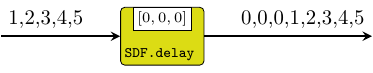Arguments

 :: ((Cons, Cons), (Prod, Prod), [a1] -> [a2] -> ([b1], [b2])) function on lists of values, tupled with consumption / production rates -> Signal a1 first input signal -> Signal a2 second input signal -> (Signal b1, Signal b2) two output signals

actor processes map combnational functions on signals and take care of synchronization between input signals. It instantiates the comb atom pattern (see comb22).

Constructors: actor[1-4][1-4].

>>> let s1 = signal [1..]
>>> let s2 = signal [1,1,1,1,1,1,1]
>>> let f [a,b,c] [d,e] = [a+d, c+e]
>>> actor21 ((3,2),2,f) s1 s2
{2,4,5,7,8,10}


Incorrect usage (not covered by doctest):

λ> actor21 ((3,2),3,f) s1 s2
*** Exception: [MoC.SDF] Wrong production## Composite

These are not-so-meaningful process constructors, but display interesting behavior nevertheless. While not commonly used or met in regular designs, these constructors are merely SDF instantiations of the ForSyDe.Atom.MoC patterns, showing that these patterns can be overloaded no matter the MoC they implement.

Arguments

 :: Signal a signal containing the initial tokens -> Signal a input signal -> Signal a output signal

Similar to the previous, but this is the raw instantiation of the delay pattern. It appends the contents of one signal at the head of another signal.

>>> let s1 = signal [0,0,0]
>>> let s2 = signal [1,2,3,4,5]
>>> delay' s1 s2
{0,0,0,1,2,3,4,5}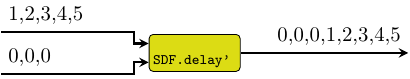Arguments

 :: ((Cons, Cons), (Prod, Prod)) -> Signal ([a1] -> [a2] -> ([b1], [b2])) function on lists of values, tupled with consumption / production rates -> Signal a1 first input signal -> Signal a2 second input signal -> (Signal b1, Signal b2) two output signals

reconfig creates an SDF adaptive process where the first signal carries functions and the other carry the arguments. It instantiates the reconfig atom pattern (see reconfig22). According to our SDF definition, the production and consumption rates need to be fixed, so they are passed as parameters to the constructor, whereas the first signal carries adaptive functions only. For the adaptive signal it only makes sense that the consumption rate is always 1.

Constructors: reconfig[1-4][1-4].

>>> let f1 a = [sum a]
>>> let f2 a = [maximum a]
>>> let sf = signal [f1,f2,f1,f2,f1,f2,f1]
>>> let s1 = signal [1..]
>>> reconfig11 (4,1) sf s1
{10,8,42,16,74,24,106}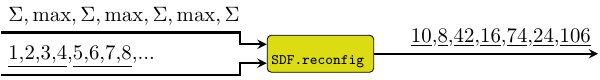Arguments

 :: ([b1], [b2]) values to be repeated -> (Signal b1, Signal b2) generated signals

A signal generator which repeats the initial tokens indefinitely. It is actually an instantiation of the stated0X constructor (check stated22).

Constructors: constant[1-4].

>>> let (s1, s2) = constant2 ([1,2,3],[2,1])
>>> takeS 7 s1
{1,2,3,1,2,3,1}
>>> takeS 5 s2
{2,1,2,1,2}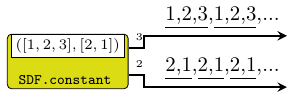Arguments

 :: ((Cons, Cons), (Prod, Prod), [b1] -> [b2] -> ([b1], [b2])) function to generate next value, tupled with consumption / production rates -> ([b1], [b2]) values of initial tokens -> (Signal b1, Signal b2) generated signals

A signal generator based on a function and a kernel value. It is actually an instantiation of the stated0X constructor (check stated22).

Constructors: generate[1-4].

>>> let f a b = ([sum a, sum a],[sum b, sum b, sum b])
>>> let (s1,s2) = generate2 ((2,3),(2,3),f) ([1,1],[2,2,2])
>>> takeS 7 s1
{1,1,2,2,4,4,8}
>>> takeS 8 s2
{2,2,2,6,6,6,18,18}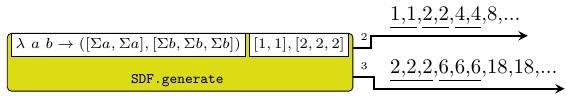Arguments

 :: ((Cons, Cons, Cons, Cons), (Prod, Prod), [b1] -> [b2] -> [a1] -> [a2] -> ([b1], [b2])) next state function, tupled with consumption / production rates -> ([b1], [b2]) initial state partitions of values -> Signal a1 first input signal -> Signal a2 second input signal -> (Signal b1, Signal b2) output signals

stated is a state machine without an output decoder. It is an instantiation of the state MoC constructor (see stated22).

Constructors: stated[1-4][1-4].

>>> let f [a] [b,c] = [a+b+c]
>>> let s = signal [1,2,3,4,5,6,7]
>>> stated11 ((1,2),1,f)  s
{1,4,11,22}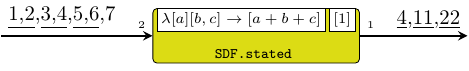Arguments

 :: ((Cons, Cons, Cons, Cons), (Prod, Prod), [b1] -> [b2] -> [a1] -> [a2] -> ([b1], [b2])) next state function, tupled with consumption / production rates -> ([b1], [b2]) initial partitions of values -> Signal a1 first input signal -> Signal a2 second input signal -> (Signal b1, Signal b2) output signals

state is a state machine without an output decoder. It is an instantiation of the stated MoC constructor (see state22).

Constructors: state[1-4][1-4].

>>> let f [a] [b,c] = [a+b+c]
>>> let s = signal [1,2,3,4,5,6,7]
>>> state11 ((1,2),1,f)  s
{4,11,22}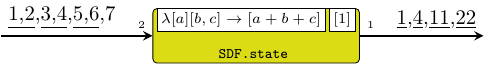Arguments

 :: ((Cons, Cons, Cons), Prod, [st] -> [a1] -> [a2] -> [st]) next state function, tupled with consumption / production rates -> (Cons, (Prod, Prod), [st] -> ([b1], [b2])) output decoder, tupled with consumption / production rates -> [st] initial state values -> Signal a1 -> Signal a2 -> (Signal b1, Signal b2)

moore processes model Moore state machines. It is an instantiation of the moore MoC constructor (see moore22).

Constructors: moore[1-4][1-4].

>>> let ns [a] [b,c] = [a+b+c]
>>> let od [a]       = [a+1,a*2]
>>> let s = signal [1,2,3,4,5,6,7]
>>> moore11 ((1,2),1,ns) (1,2,od)  s
{2,2,5,8,12,22,23,44}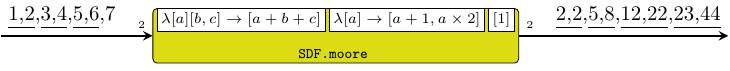Arguments

 :: ((Cons, Cons, Cons), Prod, [st] -> [a1] -> [a2] -> [st]) next state function, tupled with consumption / production rates -> ((Cons, Cons, Cons), (Prod, Prod), [st] -> [a1] -> [a2] -> ([b1], [b2])) outpt decoder, tupled with consumption / production rates -> [st] initial state values -> Signal a1 -> Signal a2 -> (Signal b1, Signal b2)

mealy processes model Mealy state machines. It is an instantiation of the mealy MoC constructor (see mealy22).

Constructors: mealy[1-4][1-4].

>>> let ns [a] [b,c] = [a+b+c]
>>> let od [a] [b]   = [a+b,a*b]
>>> let s = signal [1,2,3,4,5,6,7]
>>> mealy11 ((1,2),1,ns) ((1,1),2,od)  s
{2,1,6,8,14,33,26,88}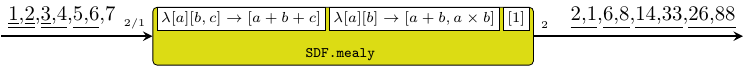## Interfaces

Arguments

 :: (Cons, Cons) consumption rate of interface process -> Signal a SDF signal -> Signal b SDF signal -> (Signal (Vector a), Signal (Vector b)) SY signals

Transforms a (set of) SDF signal(s) into the equivalent SY signal(s). The partial ordering is transformed to total ordering with respect to the firings of the interface process(es), and events consumed during one firing are grouped into vectors.

Constructors: toSY[1-4].

>>> let s = SDF.signal [1,2,3,4,5,6,7,8,9]
>>> toSY1 2 s
{<1,2>,<3,4>,<5,6>,<7,8>}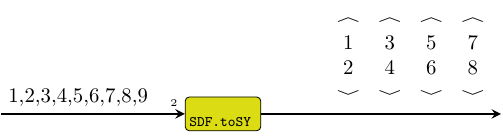Arguments

 :: Signal a SDF signal -> Signal b SDF signal -> (Signal a, Signal b) SY signals

Alternative implementation to toSY2, where the consumption rate of the interface process is 1, meaning that each SDF event has a corresponding SY event.

Constructors: toSY[1-4]'.

>>> let s = SDF.signal [1,2,3,4,5]
>>> toSY1' s
{1,2,3,4,5}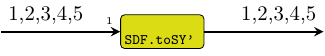Arguments

 :: Vector Cons consumption rates -> Vector (Signal a) vector of signals -> Signal (Vector a) signal of vectors

Consumes tokens from a vector of signals and merges them into a signal of vectors, with a production rate of 1. It instantiates the zipx skeleton.

>>> let s1 = SDF.signal [1,2,3,4,5]
>>> let s2 = SDF.signal [11,12,13,14,15]
>>> let v1 = V.vector [s1,s1,s2,s2]
>>> let r  = V.vector [2,1,2,1]
>>> v1
<{1,2,3,4,5},{1,2,3,4,5},{11,12,13,14,15},{11,12,13,14,15}>
>>> zipx r v1
{<1,2,1,11,12,11>,<3,4,2,13,14,12>}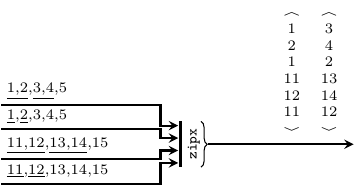Arguments

 :: Vector Prod production rates (in reverse order) -> Signal (Vector a) signal of vectors -> Vector (Signal a) vector of signals

Consumes the vectors carried by a signal with a rate of 1, and unzips them into a vector of signals based on the user provided rates. It instantiates the unzipx skeleton.

OBS: due to the recur pattern contained by unzipx, the vector of production rates needs to be provided in reverse order (see ForSyDe.Atom.Skel.Vector).

>>> let s1 = SDF.signal [1,2,3,4,5]
>>> let s2 = SDF.signal [11,12,13,14,15]
>>> let v1 = V.vector [s1,s1,s2,s2]
>>> let r  = V.vector [2,1,2,1]
>>> let sz = zipx r v1
>>> v1
<{1,2,3,4,5},{1,2,3,4,5},{11,12,13,14,15},{11,12,13,14,15}>
>>> sz
{<1,2,1,11,12,11>,<3,4,2,13,14,12>}
>>> unzipx (V.reverse r) sz
<{1,2,3,4},{1,2},{11,12,13,14},{11,12}>